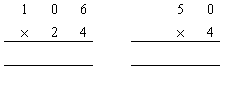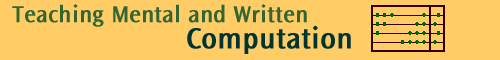Back to Multiply

# Activity: Common errors in multiplication

Mathematics teachers have to be careful to analyze written work in arithmetic so that error patterns in children's work do not go unnoticed. Careless errors can affect any question, but greater consequence are the errors caused by applying a defective algorithm all of the time. These systematic errors may go undetected because they often yield correct answers to some questions. It is important for the teacher to understand where the student is having difficulty and employ teaching strategies specific to each child's problem.

 Teaching strategies may include: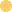leading children to see that their method is incorrect by demonstrating their answer is not possible.using calculators to check answers.orally solving problems as a group and discussing the mathematical concept applicable at each step.Using place value material such as MAB.ensuring that students are competent with each stage of the multiplication algorithm (e.g. by single digit) before moving on to the next. For more information about the stages, see Teaching algorithms for multiplication.

Given below are the written responses of some children to multiplication questions. Study the various examples to develop an understanding of the common error patterns seen among children.

# | Kim | Jane | Jolly | Sid | Tom |

Kim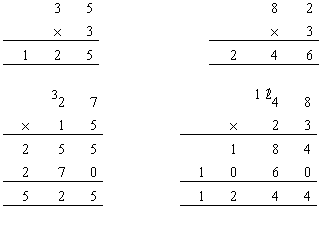Explain the error in Kim's method.

Did Kim get any question correct using this method?

Complete the following questions the way Kim would.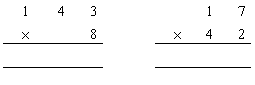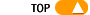Jane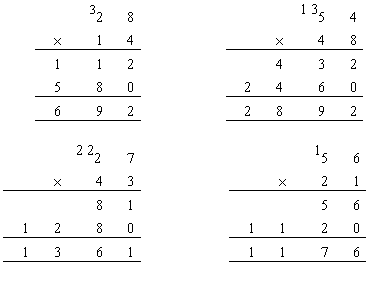Explain the error in Jane's method.

Did Jane get any question correct using this method?

Complete the following questions the way Jane would.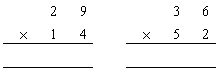Jolly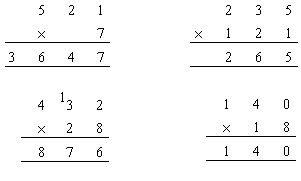Explain the error in Jolly's method.

Did Jolly get any question correct using this method?

Complete the following questions the way Jolly would.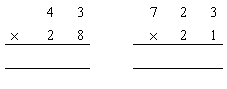SidExplain the error in Sid's method.

Did Sid get any question correct using this method?

Complete the following questions the way Sid would.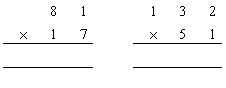Tom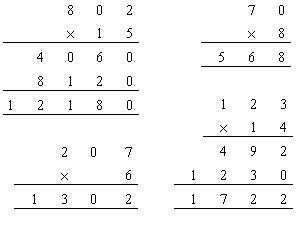Explain the error in Tom's method.

Did Tom get any question correct using this method?

Complete the following questions the way Tom would.